# WAVES WAVES waves waves waves waves 1 General

• Slides: 39WAVES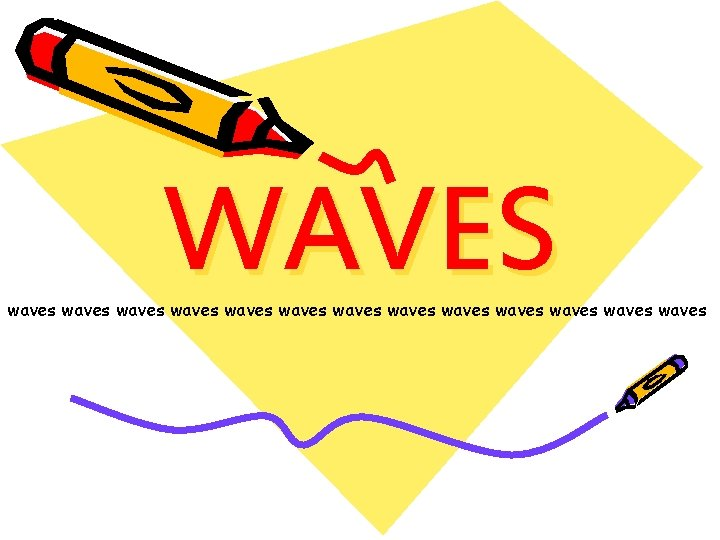WAVES waves waves waves waves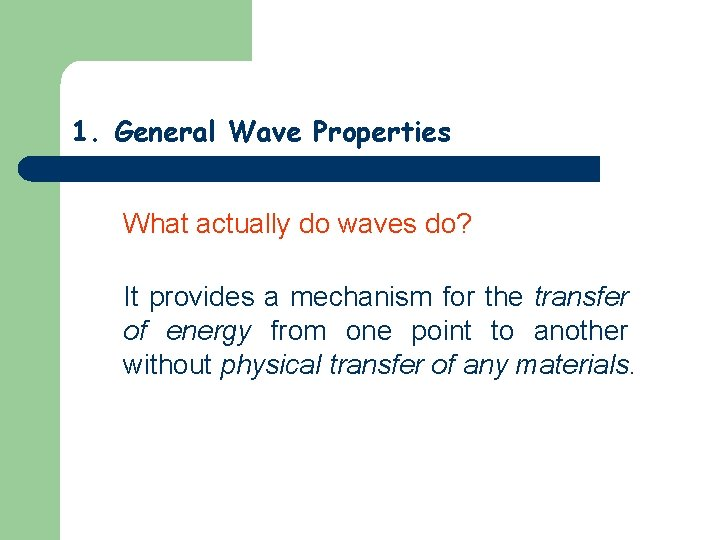1. General Wave Properties What actually do waves do? It provides a mechanism for the transfer of energy from one point to another without physical transfer of any materials.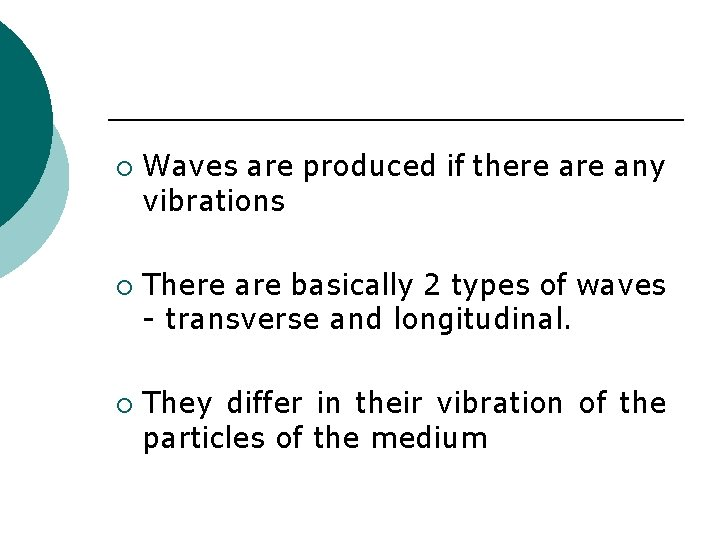¡ ¡ ¡ Waves are produced if there any vibrations There are basically 2 types of waves - transverse and longitudinal. They differ in their vibration of the particles of the medium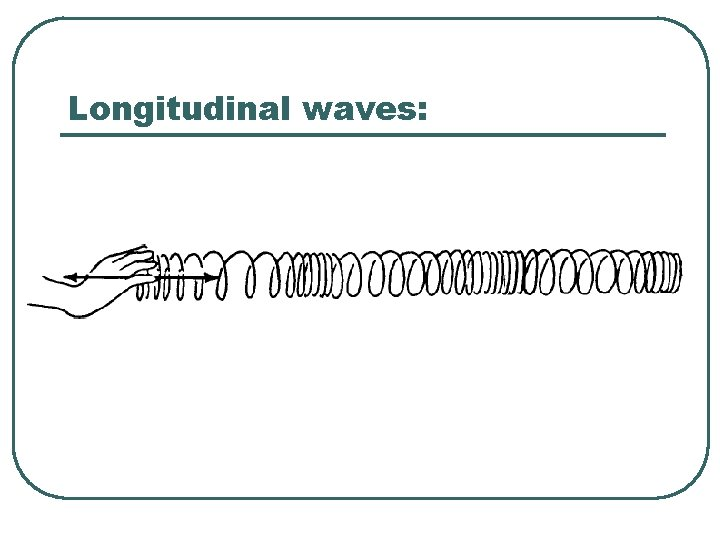Longitudinal waves: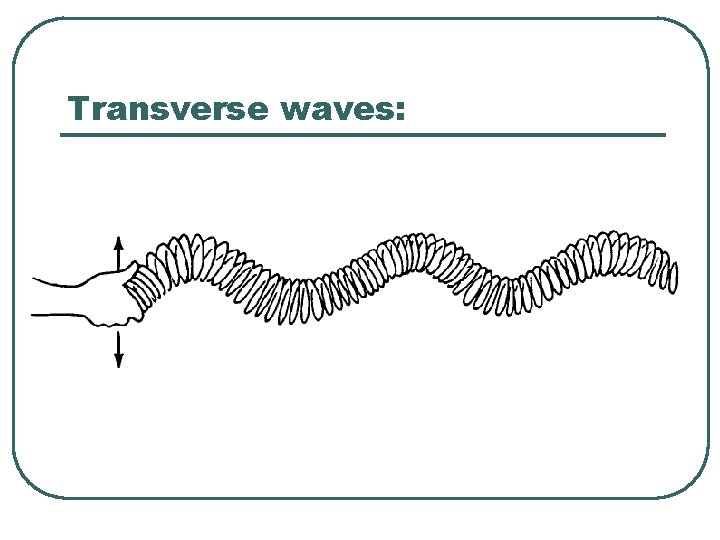Transverse waves: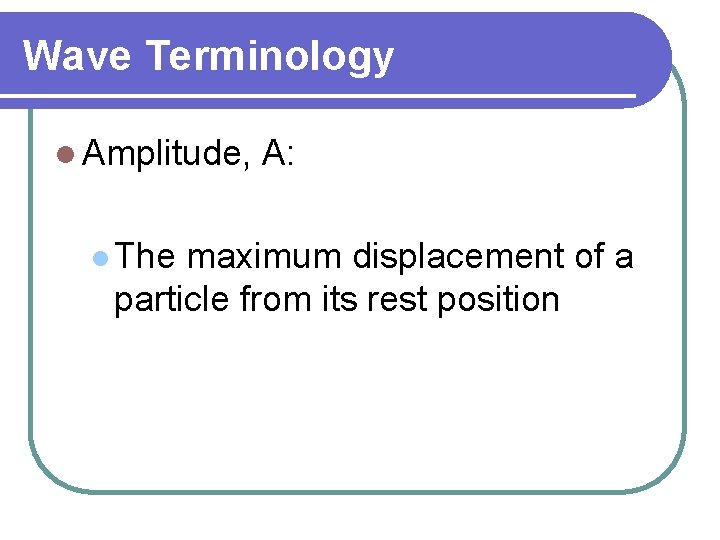Wave Terminology l Amplitude, l The A: maximum displacement of a particle from its rest positionWave length (λ) l The distance between two successive particles which are in same phase and same position It can also be defined as the distance between two successive crests or troughs for transverse waves l It can also be defined as the distance between two successive compressions or rarefactions for longitudinal waves l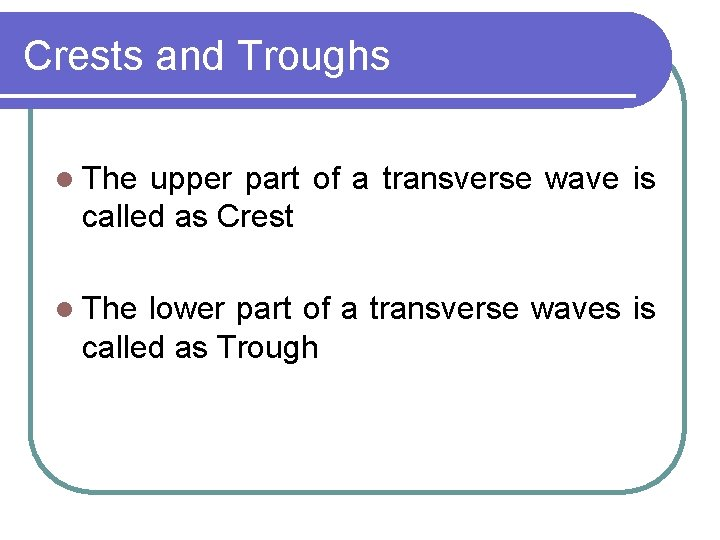Crests and Troughs l The upper part of a transverse wave is called as Crest l The lower part of a transverse waves is called as TroughPhase l The direction of movement of a particle when the waves passes is called as PhaseFrequency (f) l The number of complete oscillations made by a particle in one second l It can also be defined as the number of waves passed in one second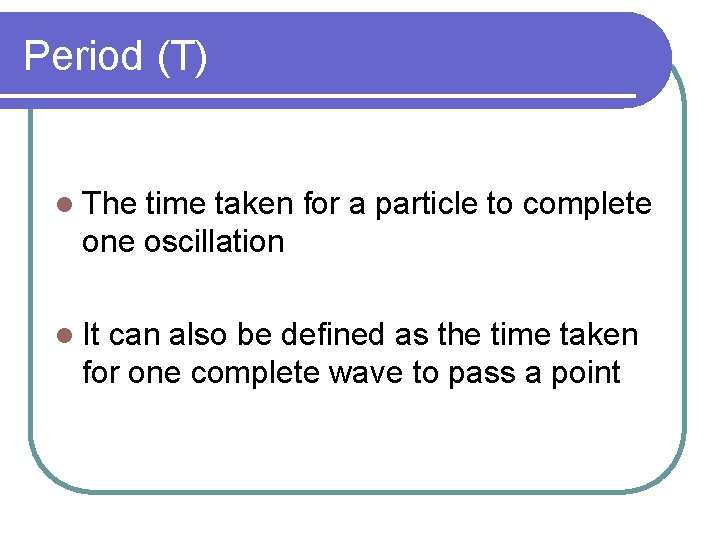Period (T) l The time taken for a particle to complete one oscillation l It can also be defined as the time taken for one complete wave to pass a point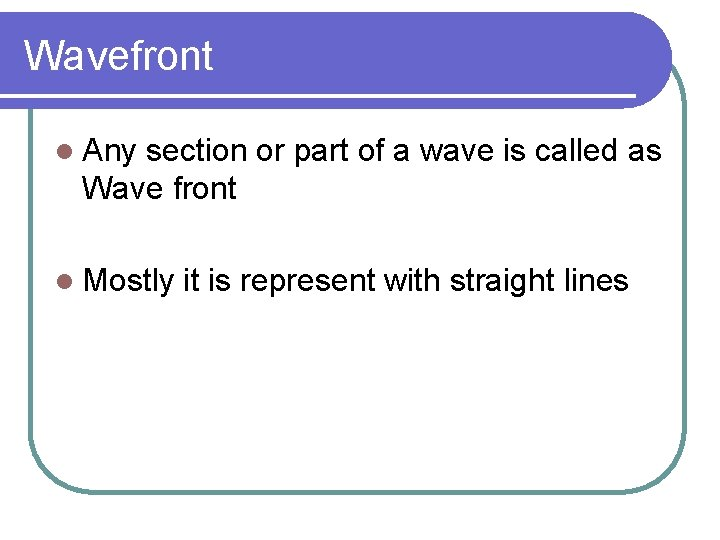Wavefront l Any section or part of a wave is called as Wave front l Mostly it is represent with straight linesQuestion ¡ Figure shows the graph of the variation of the displacement of a wave with distance along the wave at a particular time.State values for ¡ (i) the amplitude of the wave = cm ¡ (ii) the wavelength of the wave = cm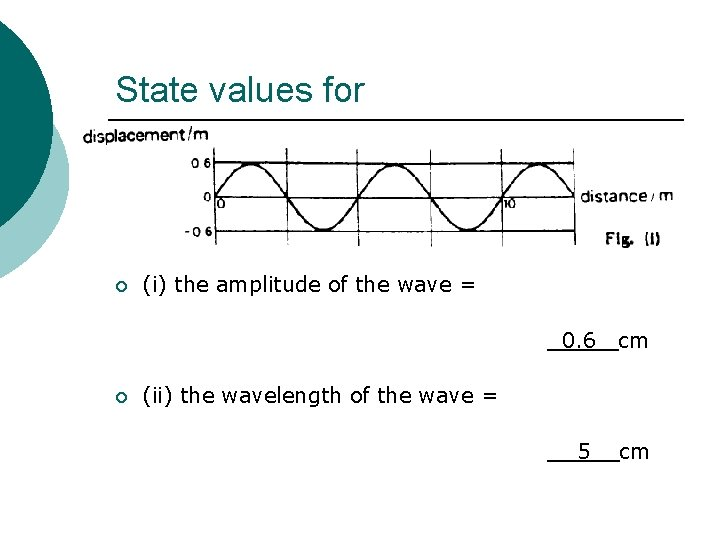State values for ¡ ¡ (i) the amplitude of the wave = 0. 6 cm 5 cm (ii) the wavelength of the wave =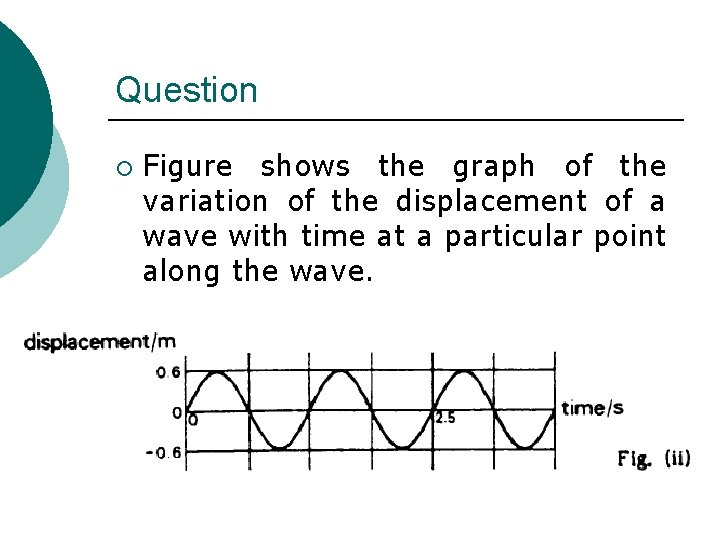Question ¡ Figure shows the graph of the variation of the displacement of a wave with time at a particular point along the wave.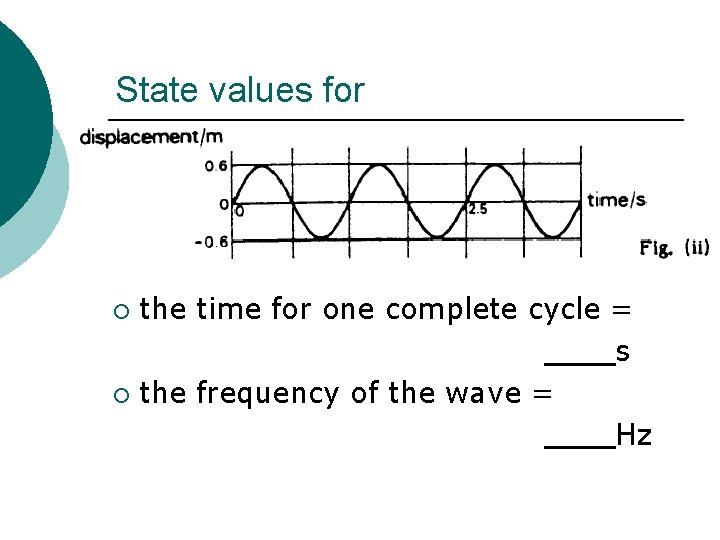State values for the time for one complete cycle = s ¡ the frequency of the wave = Hz ¡State values for the time for one complete cycle = 1. 25 s ¡ the frequency of the wave = 0. 8 Hz ¡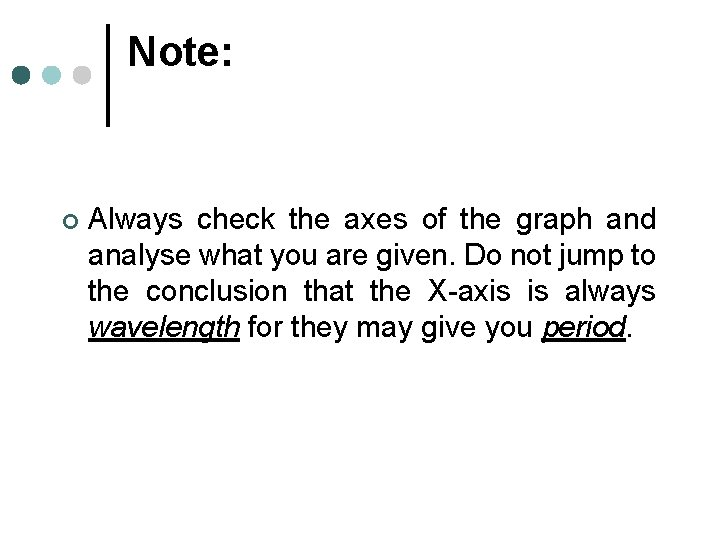Note: ¢ Always check the axes of the graph and analyse what you are given. Do not jump to the conclusion that the X-axis is always wavelength for they may give you period.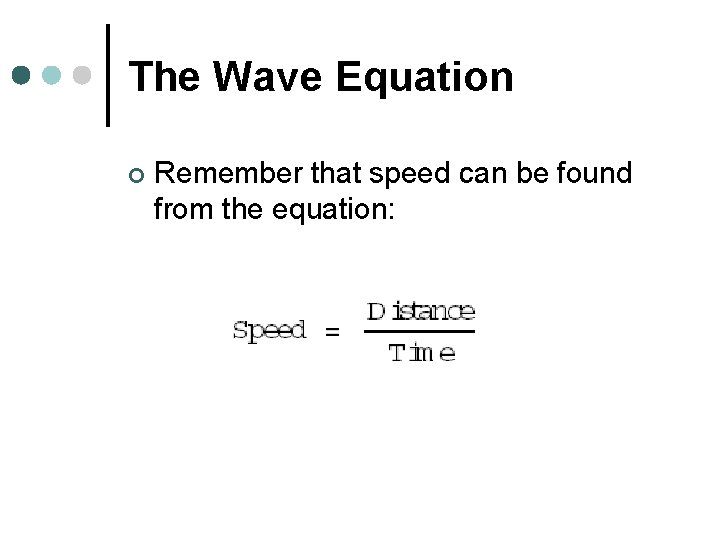The Wave Equation ¢ Remember that speed can be found from the equation:The Wave Equation For waves, But v = velocity λ = wavelength (distance) f= 1 T So v = λ T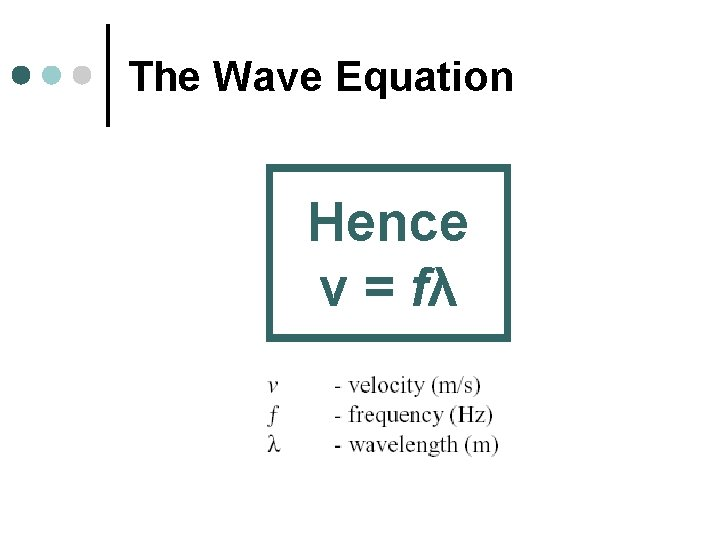The Wave Equation Hence v = fλ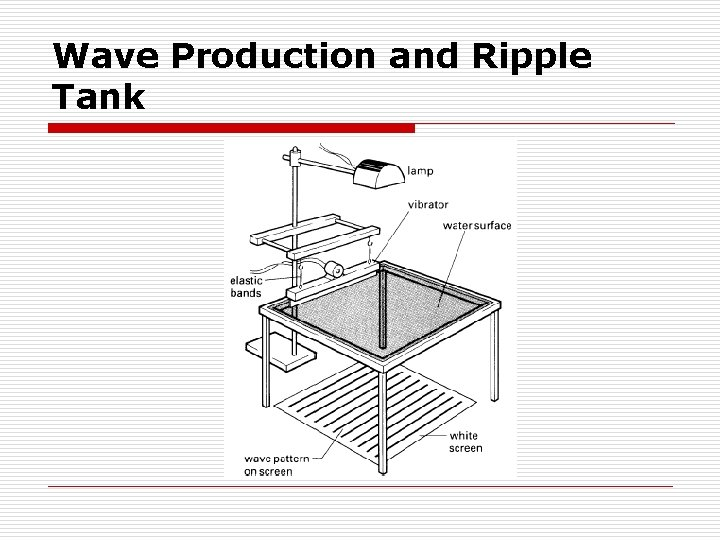Wave Production and Ripple Tank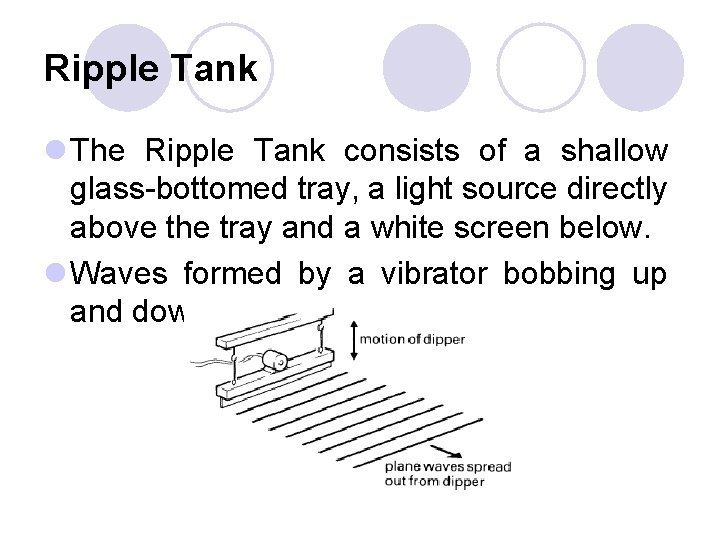Ripple Tank l The Ripple Tank consists of a shallow glass-bottomed tray, a light source directly above the tray and a white screen below. l Waves formed by a vibrator bobbing up and down.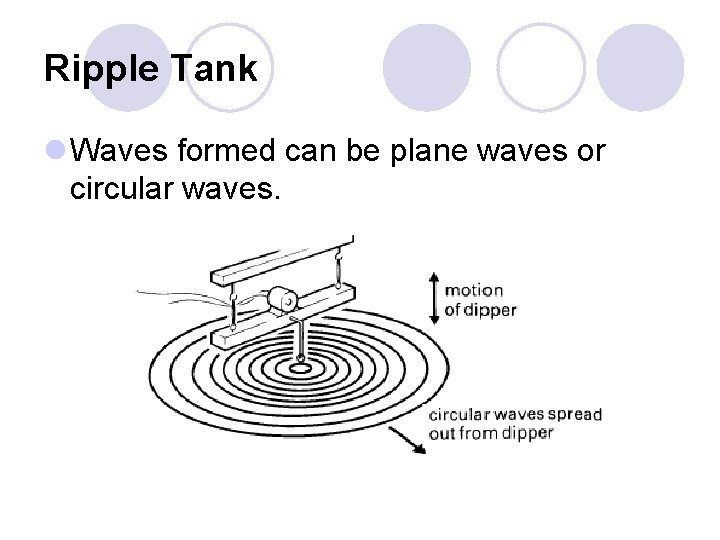Ripple Tank l Waves formed can be plane waves or circular waves.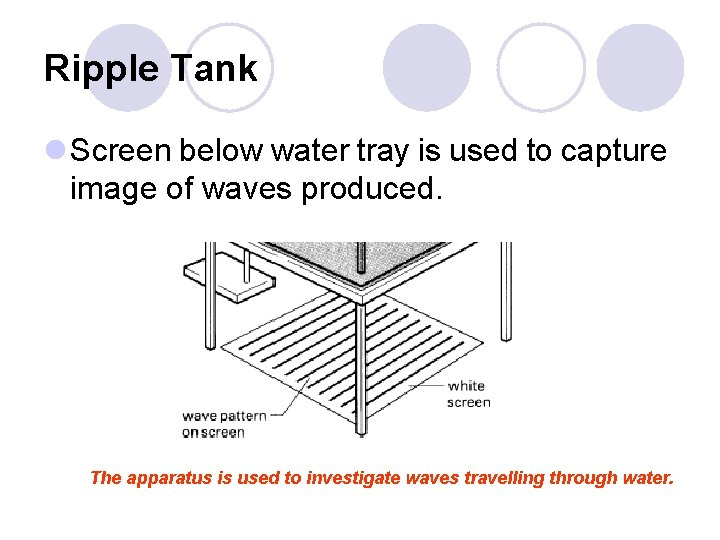Ripple Tank l Screen below water tray is used to capture image of waves produced. The apparatus is used to investigate waves travelling through water.Reflection of Waves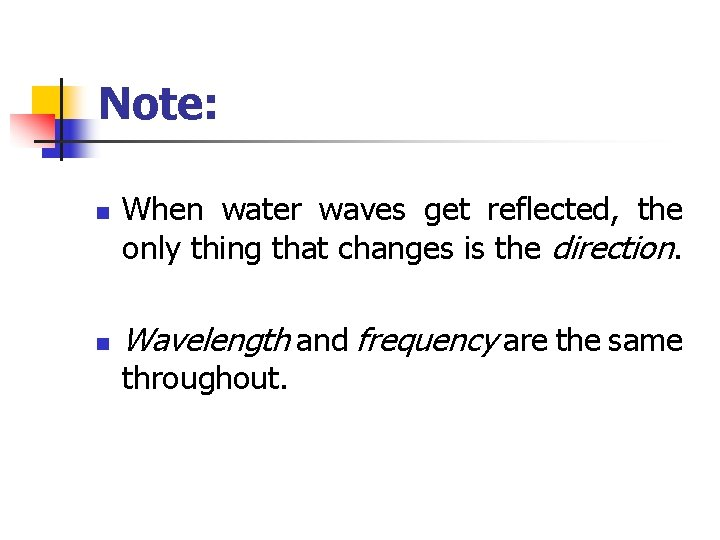Note: n n When water waves get reflected, the only thing that changes is the direction. Wavelength and frequency are the same throughout.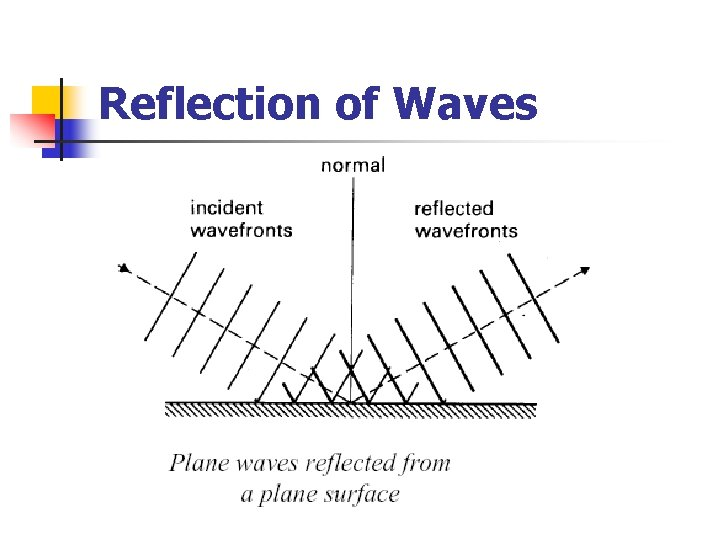Reflection of WavesReflection of WavesEffect of Water depth on WavesEffect of Water depth on WavesEffect of Water depth on Waves n In moving from deep to shallow water the water, q q q the waves will move with a slow speed, the waves’ wavelength will become shorter, the frequency of the waves will be constant.Refraction of Waves • Refraction of water waves occurs when waves move from deep water to shallow water (or vice versa). As with light it is brought about due to the different speeds of the waves passing through differing media.Refraction of Waves • We have already seen waves entering a region of shallow water will be slowed down.Refraction of Waves • Seen from the side view the change in wavelength can be seen more clearly.Refraction of Waves • In a similar way to light slowing down as it enters a different media this will result in the water waves bending towards the normal as they pass from deep to shallow water.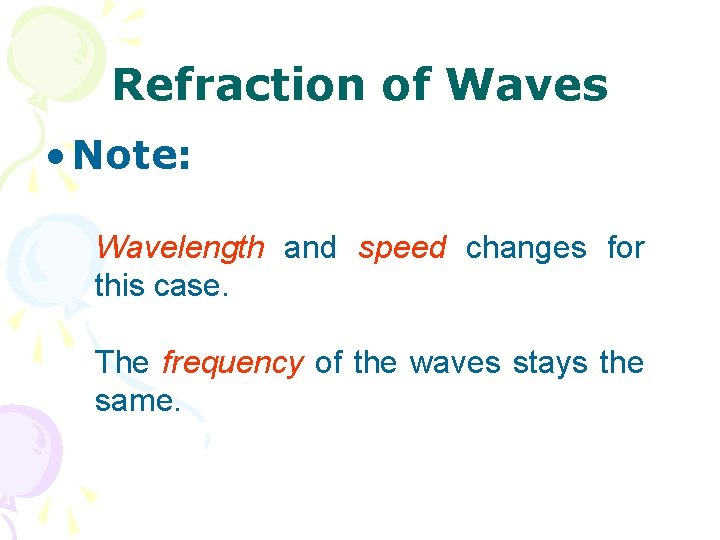Refraction of Waves • Note: Wavelength and speed changes for this case. The frequency of the waves stays the same.